## 第三次作业

1、实验代码

#include<stdio.h>
int main()
{
int x,sum;
for(x=1;x<=100;x++){
sum=sum+x;
}
printf("sum = %d",sum);
return 0;}

2、设计思路

3、流程图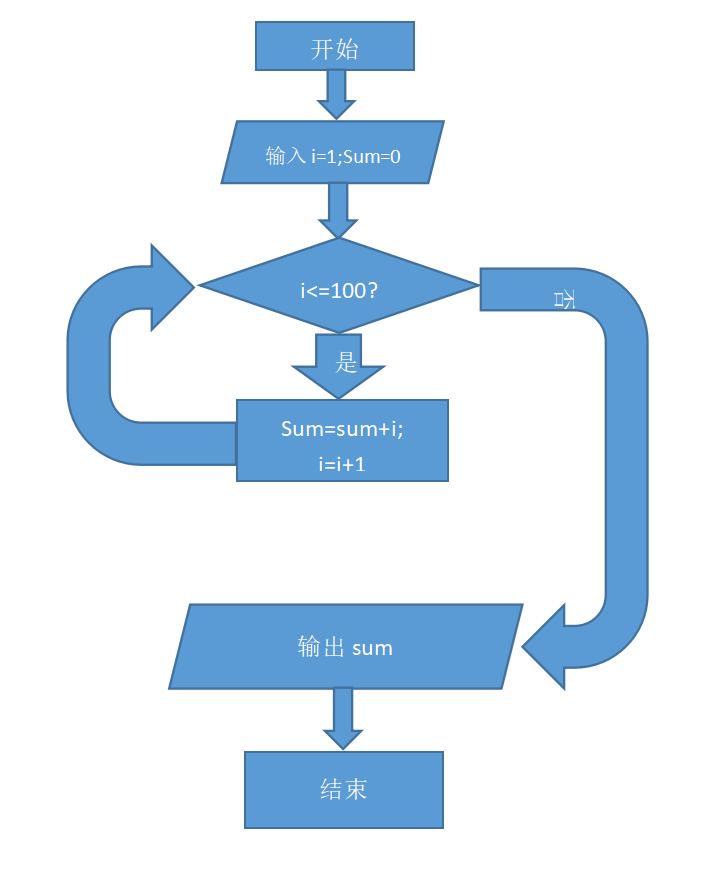4、调试过程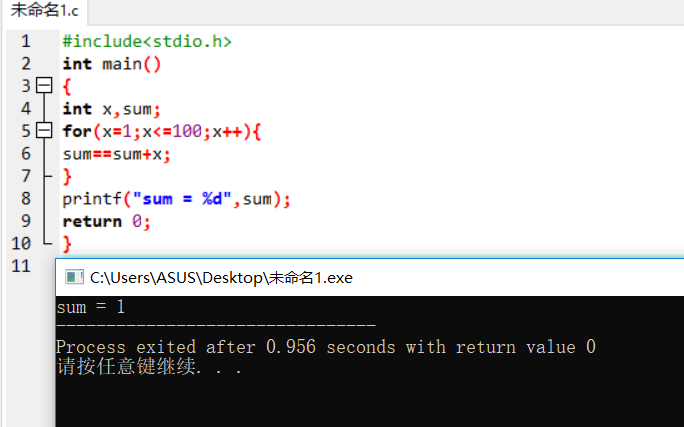1、实验代码

#include<stdio.h>
int main()
{
int N,a=1;
scanf("%d",&N);
while(N>1){
a=(a+1)*2;
N=N-1;
}
printf("%d",a);
return 0;
}

2、设计思路

1、合理运用逆向思维，由最后的1，慢慢的往前推导；

2、定义一个变量N记为天数，在定义最后桃子的个数等于一；

3、根据题意得出其关系式，应是先加一，再乘二，然后天数减一；

4、经过循环，得出结果。

3、流程图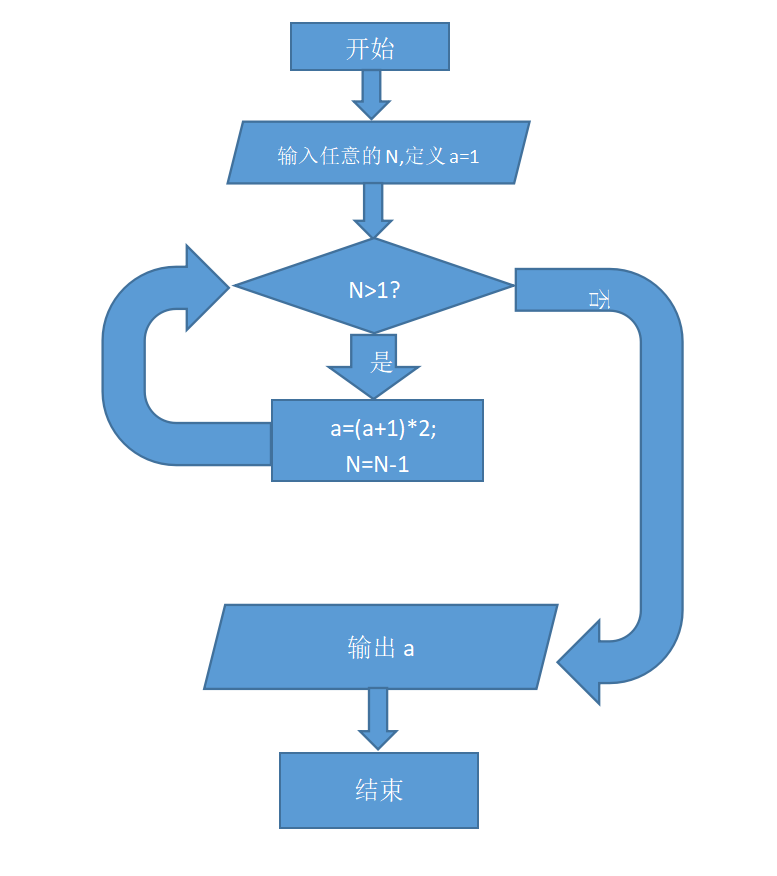4、调试过程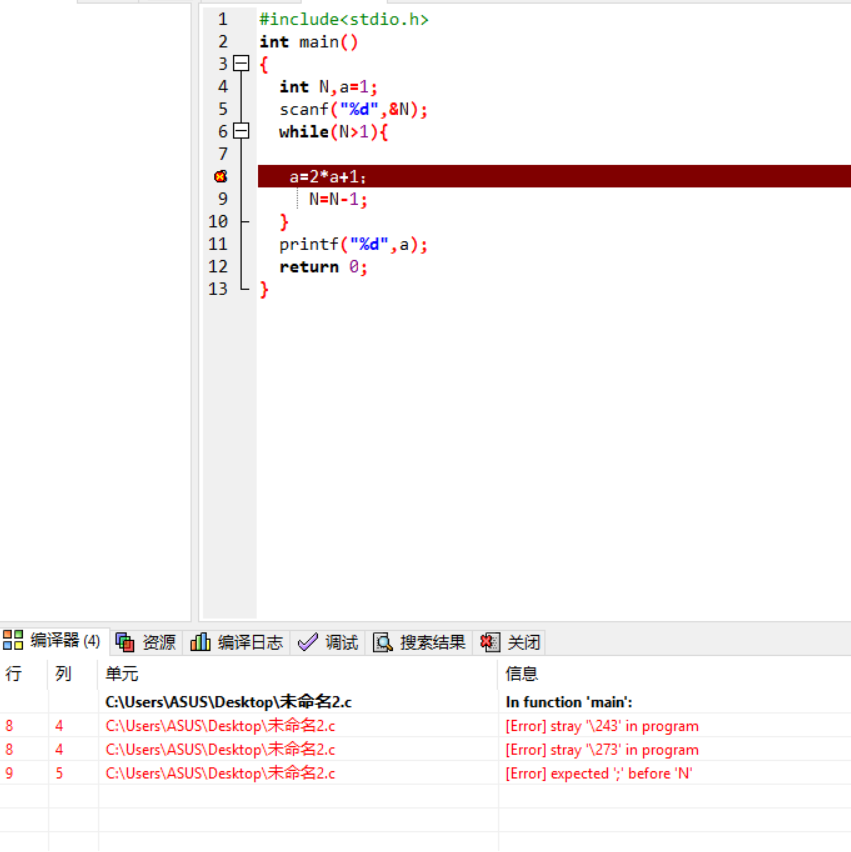1、实验代码

#include <stdio.h>

int max( int a, int b );

int main()
{
int a, b;

scanf("%d %d", &a, &b);
printf("max = %d\n", max(a, b));

return 0;
}
int max( int a, int b ){
int max;
if(a>b){
max=a;
}else max=b;
return max;
}

2、设计思路

3、流程图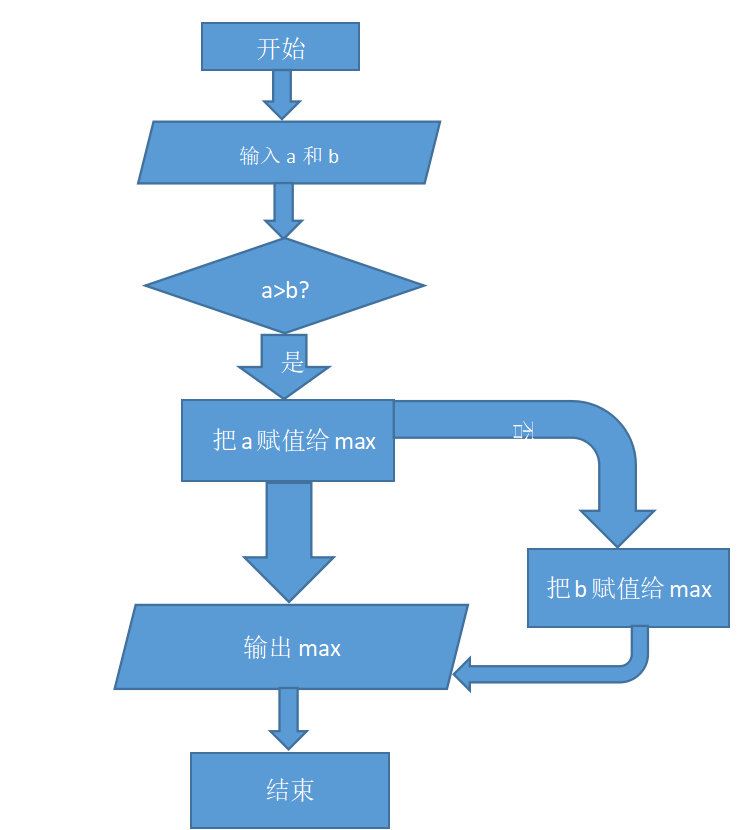4、调试过程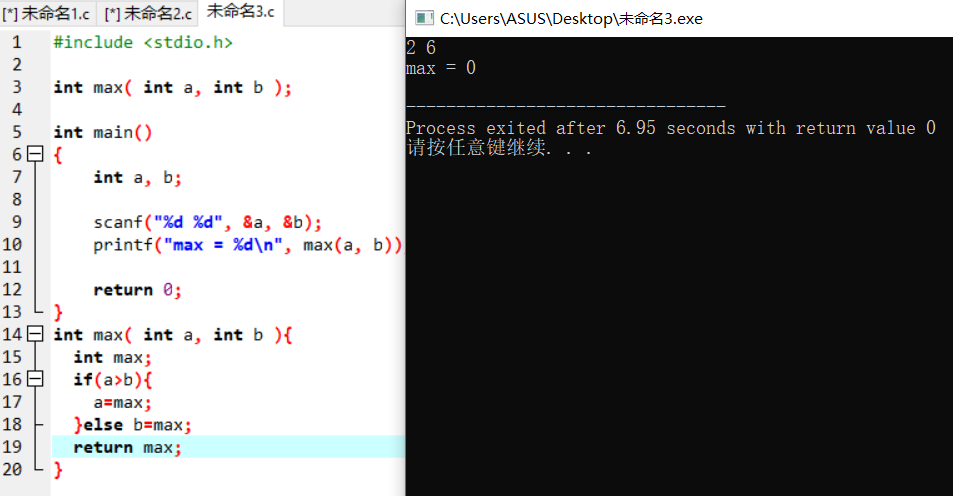程序可以运行，但输入两个不同的数后，无论是什么结果都是0；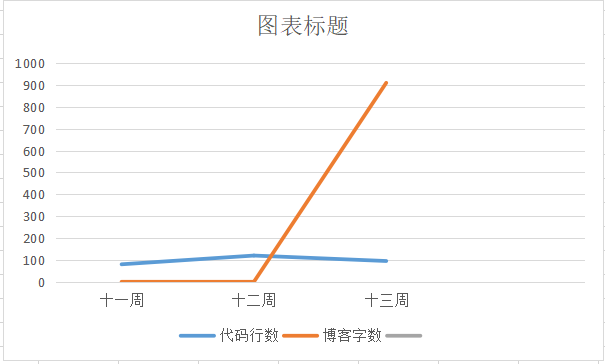posted on 2018-12-01 11:03  赵天华2018023954  阅读(162)  评论(4编辑  收藏  举报